# Managerial Economics Study Set 10

## Quiz 11 : Game Theory and Asymmetric InformationStudy FlashcardsLooking for Economics Homework Help?

## Quiz 11 :Game Theory and Asymmetric Information

Question TypeIs the prisoners' dilemma more of a problem for a one-shot or a repeated game
Free
Essay

A game is the strategic interaction between two or more players in the process of economic decision making. Each agent who makes the decision in a game is called the player. Each player has a set of choices to decide upon, these choices are called the strategies. The strategies are tied to certain outcomes called payoffs.
The one shot game is one that is played for only one period. A repeated game is played for more than one period.
The table below gives the Prisoner's dilemma game for Gray and White.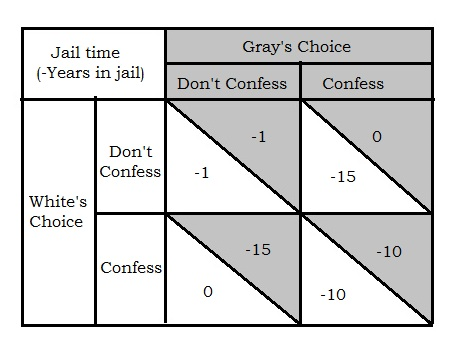Figure 1
To solve the Nash equilibrium the best response of each player is selected given the moves of the other players. If White chooses to "don't confess", it will be best for Gray to "confess" that will get him 0 year in jail than to "don't confess" which will get him 1 years in jail. On the other hand, if White chooses to "confess", it will be best for Gray to "confess" that will get him 10 years in jail than to "don't confess" which will get him 15 years in jail.
Hence, "confess" is the dominant strategy of Gray. This means no matter what strategy White choose, Gray will choose "confess"
Similarly, if Gray chooses to "don't confess", it will be best for White to "confess" that will get him 0 year in jail than to "don't confess" which will get him 1 year in jail. On the other hand, if Gray chooses to "confess", it will be best for White to "confess" that will get him 10 years in jail than to "don't confess" which will get him 15 years in jail.
Hence, "confess" is the dominant strategy of White. This means no matter what strategy Gray choose, White will choose "confess".
Hence, "confess" is the dominant strategy for both players and the Nash equilibrium occurs where both "confess" and serve 10 years in jail. Here it will be best for both to choose "don't confess" as it will give them a much less time in jail. But given the strategy of the opponent they reached an equilibrium which is not associated with the highest payoff of the players. This is the prisoner's dilemma.
The prisoner's dilemma is a one shot game. That is the prisoner's dilemma arises when a game is played for one period. If the game is repeated for more than one period, the players tend to learn from their past mistakes and their rival. There is also some opportunity that the players will engage in some bargaining and arrive at a solution which is mutually beneficial. In that case the game will not remain a prisoner's dilemma.
Therefore, the prisoner's dilemma is more of a problem for one shot game.

Tags
Choose question tagIn your answer to each part below, include references to specific games discussed in the chapter as appropriate. a. If a game has a dominant strategy equilibrium, does it have a Nash equilibrium b. If a game has a Nash equilibrium, does it have a dominant strategy equilibrium c. If one firm has a dominant strategy, can another firm take advantage of that fact in deciding on its optimal strategy d. Can a game have more than one dominant strategy equilibrium Can a game have more than one Nash equilibrium
Free
Essay

A game is the strategic interaction between two or more players in the process of economic decision making. Each agent who makes the decision in a game is called the player. Each player has a set of choices to decide upon, these choices are called the strategies. The strategies are tied to certain outcomes called payoffs.
The Nash equilibrium of the game occurs when both the player takes their best strategies in response to the strategies of the other players. The prisoner's dilemma is a situation where the players choses a Nash equilibrium that is not associated with the highest payoff of the players.
The move that the player choses no matter what the other player do is called the dominant strategy. The move that a player never plays no matter what the other player do is called the dominated strategy. The equilibrium where each player plays their dominant strategies is called the dominant strategy equilibrium.
a. If each player chooses their dominant strategy, the game has dominant strategy equilibrium. This can be Nash equilibrium is the dominant strategy equilibrium if the optimum move of each player given the moves of other player is their dominant strategy.
This is given by the "prisoner's dilemma game". The table below gives the game between gray and white over the choice to confess or don't confess.
.Figure
To solve the Nash equilibrium the best response of each player is selected given the moves of the other players. If White chooses to "don't confess", it will be best for Gray to "confess" that will get him 0 year in jail than to "don't confess" which will get him 1 years in jail. On the other hand, if White chooses to "confess", it will be best for Gray to "confess" that will get him 10 years in jail than to "don't confess" which will get him 15 years in jail.
Hence, "confess" is the dominant strategy of Gray. This means no matter what strategy White choose, Gray will choose "confess"
Similarly, if Gray chooses to "don't confess", it will be best for White to "confess" that will get him 0 year in jail than to "don't confess" which will get him 1 year in jail. On the other hand, if Gray chooses to "confess", it will be best for White to "confess" that will get him 10 years in jail than to "don't confess" which will get him 15 years in jail.
Hence, "confess" is the dominant strategy of White. This means no matter what strategy Gray choose, White will choose "confess".
Hence, "confess" is the dominant strategy for both players and the Nash equilibrium occurs where both "confess" and serve 10 years in jail. Here it will be best for both to choose "don't confess" as it will give them a much less time in jail. But given the strategy of the opponent they reached an equilibrium which is not associated with the highest payoff of the players. This is the prisoner's dilemma.
Here each player plays their dominant strategy that is the best response given the moves of the other player. Therefore, the equilibrium here is the dominant strategy equilibrium as well as the Nash equilibrium.
b. Nash equilibrium on the other hand may not be dominant strategy equilibrium. This is given by the game below between white and gray :
The table below gives the profits for White and Gray.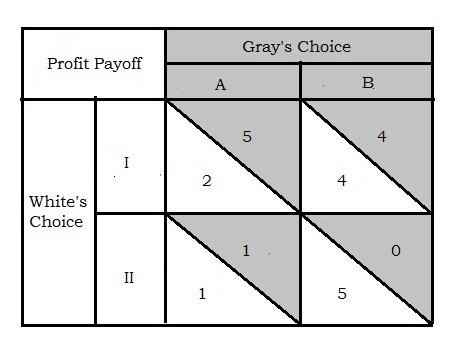Figure 2
In this game, if White chooses to "I", it will be best for Gray to choose "A" that will get him 5 units of profit than to "B" which will get him 4. On the other hand, if White chooses to play "II", it will be best for Gray to "A" that will get him I unit of profit than to "B" which will get him 0.
Hence, "A" is the dominant strategy of Gray. This means no matter what strategy White choose, Gray will choose "A"
Similarly, if Gray chooses to "A", it will be best for White to choose "I" that will get him 2 units of profit than to "II" which will get him 1. On the other hand, if Gray chooses "B", it will be best for White to choose "II" that will get him 5 units of profit than to choose "I" which will get him 4.
Hence, there is no dominant strategy of White. This means the white will act according to the action of gray. Now gray will always chose A. Hence the equilibrium of the game occurs at white choosing "I" and gray choosing A. The profits will be [2, 5]. This is Nash equilibrium. As white does not have a dominant strategy, the equilibrium is not dominant strategy equilibrium.
c. If a player has a dominant strategy and the other players do not, the player with no dominant strategy can take advantage of the situation to evoke threat and promises to alter the equilibrium of a game.
In the game in figure 2, white can threat Gray to declaring to choose II, if gray chooses A. The white can also promise to choose I if gray chooses B. In this case, gray is facing a threat of losing 4 units of profit if white choose II in case gray's strategy A. On the other hand, gray will lose 1 unit of profit by choosing B as white choose I.
In this situation gray will chose B and gray will stick to his promise and choose I. The equilibrium strategies of White and Gray are I and B respectively. The threat in this case, proves to be profit maximizing for white. This is a credible threat and it is rational for white to declare a credible threat to maximize the outcome of the game.
d. Each player's dominant strategy is one that the player plays no matter what the opponent choses to do. Therefore, each player has only one dominant strategy and there can be only one dominant strategy equilibrium in a game.
On the other hand, a game can have more than one Nash equilibrium. This is explained by the game below: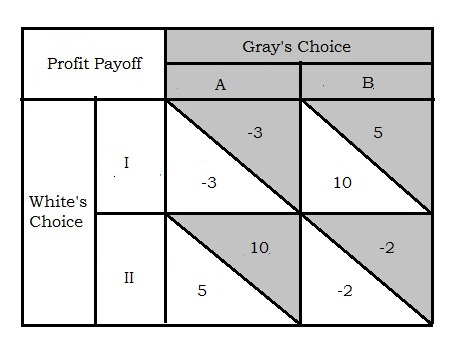Figure 3
In this game, if White chooses "I", it will be best for Gray to choose "B" that will get him 5 units of profit than to "A" which will get him -3(loss). On the other hand, if White chooses "II", it will be best for Gray to choose "A" that will get him 10 units of profit than to "B" which will get him -2.
Hence, there is no dominant strategy for Gray.
Similarly, if Gray chooses to play "A"; it will be best for White to choose "II" that will get him 5 units of profit than to "I" which will get him -3. On the other hand, if Gray
chooses "B", it will be best for White to choose "I" that will get him 10 units of profit than to choose "II" which will get him -2.
Hence, there is no dominant strategy of White. This means the white will act according to the action of gray. Now gray does not have a dominant strategy either. Hence, the equilibrium of the game occurs according to simultaneous choice of each player. If white chooses "I"; gray will choose "A". On the other hand, if white chooses "II"; gray will choose "B". Therefore, there will be two equilibria at IA and IIB. Both of them are Nash equilibrium.

Tags
Choose question tagWhy are focal points important for firms involved in tacit bargaining What is the key skill for a tacit bargainer
Free
Essay

A game is the strategic interaction between two or more players in the process of economic decision making. Each agent who makes the decision in a game is called the player. Each player has a set of choices to decide upon, these choices are called the strategies. The strategies are tied to certain outcomes called payoffs.
The bargaining in a game is either explicit or tacit. If the players can openly bargain for outcomes and terms of a game it is called implicit bargaining. If implicit bargaining is not possible, often because of legal restrictions, the bargaining is implied by the action taken by the players. This is called the tacit bargaining.
In economics situations implicit bargaining is illegal by the antitrust law. The firms cannot come to legal contract to restrict output, fix price or define geographic border of markets. If the implicit bargaining is not an option, the firms often engage in tacit bargaining. In tacit bargaining the firms cannot communicate regarding the strategies. The players of the game do not know what the other player will do given the choices. In this situation, it is important to identify the obvious, unambiguous, and conspicuous choices that will make the choice of other players predictable to each player. These obvious, unambiguous, and conspicuous choices are the focal points of a game.
In a non-cooperative game, the players cannot engage in implicit bargaining. In this case there is only tacit bargaining. Therefore, to reach the equilibrium of the game it is important to identify the focal points of the game. This makes the moves of the opponent predictable and helps each player to reach mutual beneficial outcome.

Tags
Choose question tagWhat attribute about a war makes it a limited war How does the concept of limited war relate to oligopolistic rivalry
Essay
Tags
Choose question tagConsider a small town that is served by two grocery stores, White and Gray. Each store must decide whether it will remain open on Sundays or whether it will close on that day. If both stores decide to close, then each has monthly profits of $21,000. However, if one is open and the other is closed, the open one has profits of$25,000 and the closed one has profits of $17,000. If both remain open on Sundays, then each has profits of$20,000. a. Why might profits be lower if both are open on Sundays than if both are closed on Sundays b. Place payouts in the appropriate cells of the payoff matrix shown below. c. Does either firm have a dominant strategy Is there a dominant strategy equilibrium Is this strategy joint profit maximizing d. Is the equilibrium discussed in part c likely to be stable over time In particular, what might firms do to alter this outcome e. Is this an example of a prisoners' dilemma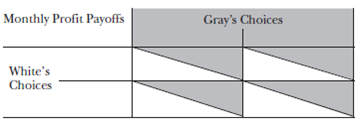Essay
Tags
Choose question tagIs a prisoners' dilemma game a zero sum or variable sum game
Essay
Tags
Choose question tagWhy are focal points important for noncooperative games
Essay
Tags
Choose question tagAssume that one-third of all used cars are lemons. If the supply of good used cars is upward sloping, then it may be the case that the blended price is lower than the reservation price for some good used car owners. Such owners would not sell. Let G represent the fraction of good used car owners who actually sell their good used car, 0 G 1. Parts a to d of this problem examine the implications of selling only some good used cars on the portion of lemons sold. a. If all good cars are sold ( G =1.0), what fraction of all used cars are sold What is the fraction of sold cars that are lemons b. If half the good cars are sold, G =0.5, what fraction of all used cars are sold What is the fraction of sold cars that are lemons c. If one-quarter of the good cars are sold, G =0.25, what fraction of all used cars are sold What is the fraction of sold cars that are lemons d. If a general portion G is sold, what fraction of all used cars are sold What are the fraction of sold cars that are lemons Make sure that your algebraic answers are consistent with your specific answers in parts a to c. Suppose the perfect information prices for lemons and cherries are P L = $5,000 and P H =$10,000. Consider how good used car owners view their cars. Suppose no owner would be willing to sell at a price of $6,000, and all good used car owners would be willing to sell at a price of$9,000. (This contrasts with Problem, where we assumed that all would sell at a price of $8,000.) If we assume that good used car owners are uniformly distributed between these upper and lower bounds, then we can describe the supply curve as being linear between these two endpoints. We can then write the inverse supply of good used cars as a function of G , the fraction of good used cars sold, as: P S ( G )=6,000 1 3,000 G. In this context, P S denotes the price at which good used car sellers are willing to sell their car. Suppose the prices in each segment under perfect information are as shown in the table below.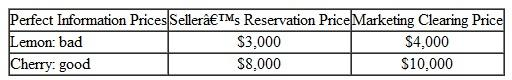A reservation price is the lowest price a seller will accept. Also assume that buyers are risk- neutral and therefore are interested only in the average quality of the cars sold in the used car market. The lemons model assumes an equal number of lemons and cherries in the initial analysis in order to simplify calculations. Consider more generally what happens based on different percentages of lemons in the market. a. What percentage of lemons and cherries are sold if the price is above the cherry seller's reservation price of$8,000 What percentage of lemons and cherries are sold if the price is below the cherry seller's reservation price of $8,000 b. Suppose 10 percent of used cars are lemons. What will be the market-clearing price given asymmetric information What portion of used cars sold will be lemons c. Suppose 25 percent of used cars are lemons. What will be the market-clearing price given asymmetric information What portion of used cars sold will be lemons d. Suppose 50 percent of used cars are lemons. What will be the market-clearing price given asymmetric information What portion of used cars sold will be lemons e. Given the reservation and market-clearing prices in the table above, what is the highest proportion of lemons in the used car market, s , that will produce a market equilibrium in which all used cars are sold What happens if the proportion of lemons in the used car market is higher than s f. Suppose that, instead of the good used car owners having a reservation price of$8,000, they had a reservation price of $7,000 but all other prices are as described in the table in Problem 6. How would your answer to part e change in this instance Problems 7 and 8 introduce the more realistic assumption that supply is upward sloping. The important segment in this instance is the high-quality segment of the market because the low-quality segment will certainly be willing to unload their lemons given the blended price that results due to the asymmetric information. As a result, we assume that all lemons will be sold given a market with asymmetric information; the question is, How many of the cherries will be sold under these conditions Reader's note : Problem 7 is difficult, even with the hints. You may wish to consider assigning it in two parts: parts a to d on one day, then go over the answers so that student can then solve parts e to g. e. Given that one-third of used cars are lemons and the full information prices of P L =$5,000 and P H = $10,000, will all used cars be sold in this situation f. If the fraction G of good used cars is sold, then what price will clear the market Write this as P B ( G ). In this context, P B denotes the price that buyers are willing to pay. Hint : It is easy to derive an equation for this price given the answer you derived in part d. g. Use the equation from part f and the equation for inverse supply to determine the market- clearing price in this market. What portion of good used cars is sold What portion of the cars sold are lemons Hint : You will have to factor a quadratic equation or use the quadratic formula to derive this answer. Essay Answer: Tags Choose question tagIs a prisoners' dilemma game more of a problem for cooperative or noncooperative games Essay Answer: Tags Choose question tagIs it easier for a rational or irrational person to make a credible threat Essay Answer: Tags Choose question tagConsider another small town that is served by two grocery stores, White and Gray. Each store must decide whether it will remain open on Sunday or whether it will close on that day. Monthly payoffs for each strategy pair are as shown in the table below.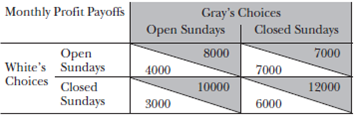a. Which firm is the most profitable in this market b. Does the firm you identified in part a have a dominant strategy c. What should this firm do Explain your answer. d. Is this an example of a prisoners' dilemma Essay Answer: Tags Choose question tagAn assertion is made in the lemons discussion in this chapter that demand may well be backward bending in a market in which quality is unobservable prior to purchase. Consider the following model: demand, X , is a function of price, P , and average quality, A. In particular, X ( P, A ) = 16000 • P 2 A 4. a. What type of demand function is this What is the price elasticity of demand and the quality elasticity of demand Average quality depends on price: A higher price in the market will create a higher average quality level in the market. Let A ( P ) = 100 • ( P /[ P + 10,000]). b. What is average quality at P =$0, $5,000,$10,000, $15,000, and$20,000 c. Based on your answer to part b, what can you say about the shape of the average quality function In particular, fill in the following sentence. Average quality _____________ at a(n) ______________ rate as price increases. d. What is demand X at P = $5,000,$10,000, $15,000, and$20,000 e. Based on your answer to part d, demand must become backward bending at a price somewhere in what range of prices In particular, fill in the following sentence. The price must be more than _________ and less than _________ at the point where demand turns from being upward sloping to downward sloping (as price increases). f. This part of Problem 9 requires calculus. Determine the price at which demand turns from being upward sloping to downward sloping (as price increases). Hint : Apply the total derivative discussed in footnote 9. g. This part of Problem 9 requires Excel, but you need not complete part f. Create two graphs: (1) average quality as a function of price in ( P, A ) space, and (2) demand as a function of price in ( X, P ) space. Make sure your average quality and demand functions have the shapes and hit the ( P, A ) and ( X, P ) values you derived in parts a to e above.
Essay
Tags
Choose question tagSuppose that a firm finds that its low-productivity workers have a marginal revenue product of $21,000 per year and that its high-productivity workers have a marginal revenue product of$35,000 per year. The cost of receiving a year's worth of higher education is $4,000 for a low-productivity worker and$3,200 for a high-productivity worker. (This cost can be thought of as repayment on loans that have been incurred in the process of obtaining the education.) The firm plans to offer a wage of $21,000 to workers without higher education and$35,000 to workers who attain a "certain" level of higher education. a. If this firm uses this pair of wage offers and wishes to create a separating equilibrium using education as a separating device, how many years of education should be required to receive the higher wage b. Why does education work as a signal in this instance c. Does education increase the marginal productivity of workers in this instance
Essay
Tags
Choose question tagThe responses to Question 9 provide an example of what game theory concept
Essay
Tags
Choose question tagWhy must much of the bargaining in economic situations be done on a tacit basis
Essay
Tags
Choose question tagWhy is it particularly important to be the party who sets the agenda in situations involving tacit negotiation
Essay
Tags
Choose question tagTo answer Problem 8, complete Problem first. Suppose that all of the information from Problem 7 holds except that the portion of lemons in the population is one-half rather than one-third. In this instance, prove that no good used cars will be offered for sale in the market equilibrium. Hints: This change affects the equation derived in Problem 7d. Thus, it also changes the weighed average price function in Problem 7f. Once these altered equations are obtained, one need only show that no portion of good used cars sold, G , satisfies the equality required by Problem 7g. Problem Assume that one-third of all used cars are lemons. If the supply of good used cars is upward sloping, then it may be the case that the blended price is lower than the reservation price for some good used car owners. Such owners would not sell. Let G represent the fraction of good used car owners who actually sell their good used car, 0 G 1. Parts a to d of this problem examine the implications of selling only some good used cars on the portion of lemons sold. a. If all good cars are sold ( G =1.0), what fraction of all used cars are sold What is the fraction of sold cars that are lemons b. If half the good cars are sold, G =0.5, what fraction of all used cars are sold What is the fraction of sold cars that are lemons c. If one-quarter of the good cars are sold, G =0.25, what fraction of all used cars are sold What is the fraction of sold cars that are lemons d. If a general portion G is sold, what fraction of all used cars are sold What are the fraction of sold cars that are lemons Make sure that your algebraic answers are consistent with your specific answers in parts a to c. Suppose the perfect information prices for lemons and cherries are P L = $5,000 and P H =$10,000. Consider how good used car owners view their cars. Suppose no owner would be willing to sell at a price of $6,000, and all good used car owners would be willing to sell at a price of$9,000. (This contrasts with Problem 6, where we assumed that all would sell at a price of $8,000.) If we assume that good used car owners are uniformly distributed between these upper and lower bounds, then we can describe the supply curve as being linear between these two endpoints. We can then write the inverse supply of good used cars as a function of G , the fraction of good used cars sold, as: P S ( G )=6,000 1 3,000 G. In this context, P S denotes the price at which good used car sellers are willing to sell their car. e. Given that one-third of used cars are lemons and the full information prices of P L =$5,000 and P H = $10,000, will all used cars be sold in this situation f. If the fraction G of good used cars is sold, then what price will clear the market Write this as P B ( G ). In this context, P B denotes the price that buyers are willing to pay. Hint : It is easy to derive an equation for this price given the answer you derived in part d. g. Use the equation from part f and the equation for inverse supply to determine the market- clearing price in this market. What portion of good used cars is sold What portion of the cars sold are lemons Hint : You will have to factor a quadratic equation or use the quadratic formula to derive this answer. Essay Answer: Tags Choose question tagSuppose you are asked to write down the name of a single card in a standard deck of playing cards. You cannot ask your neighbor what he or she is writing down, but you wish to write down the same card. What card would you write down Essay Answer: Tags Choose question tagSuppose the prices in each segment under perfect information are as shown in the table below.A reservation price is the lowest price a seller will accept. Also assume that buyers are risk- neutral and therefore are interested only in the average quality of the cars sold in the used car market. The lemons model assumes an equal number of lemons and cherries in the initial analysis in order to simplify calculations. Consider more generally what happens based on different percentages of lemons in the market. a. What percentage of lemons and cherries are sold if the price is above the cherry seller's reservation price of$8,000 What percentage of lemons and cherries are sold if the price is below the cherry seller's reservation price of $8,000 b. Suppose 10 percent of used cars are lemons. What will be the market-clearing price given asymmetric information What portion of used cars sold will be lemons c. Suppose 25 percent of used cars are lemons. What will be the market-clearing price given asymmetric information What portion of used cars sold will be lemons d. Suppose 50 percent of used cars are lemons. What will be the market-clearing price given asymmetric information What portion of used cars sold will be lemons e. Given the reservation and market-clearing prices in the table above, what is the highest proportion of lemons in the used car market, s , that will produce a market equilibrium in which all used cars are sold What happens if the proportion of lemons in the used car market is higher than s f. Suppose that, instead of the good used car owners having a reservation price of$8,000, they had a reservation price of $7,000 but all other prices are as described in the table in Problem 6. How would your answer to part e change in this instance Problems introduce the more realistic assumption that supply is upward sloping. The important segment in this instance is the high-quality segment of the market because the low-quality segment will certainly be willing to unload their lemons given the blended price that results due to the asymmetric information. As a result, we assume that all lemons will be sold given a market with asymmetric information; the question is, How many of the cherries will be sold under these conditions Reader's note : Problem 7 is difficult, even with the hints. You may wish to consider assigning it in two parts: parts a to d on one day, then go over the answers so that student can then solve parts e to g. Problem Assume that one-third of all used cars are lemons. If the supply of good used cars is upward sloping, then it may be the case that the blended price is lower than the reservation price for some good used car owners. Such owners would not sell. Let G represent the fraction of good used car owners who actually sell their good used car, 0 G 1. Parts a to d of this problem examine the implications of selling only some good used cars on the portion of lemons sold. a. If all good cars are sold ( G =1.0), what fraction of all used cars are sold What is the fraction of sold cars that are lemons b. If half the good cars are sold, G =0.5, what fraction of all used cars are sold What is the fraction of sold cars that are lemons c. If one-quarter of the good cars are sold, G =0.25, what fraction of all used cars are sold What is the fraction of sold cars that are lemons d. If a general portion G is sold, what fraction of all used cars are sold What are the fraction of sold cars that are lemons Make sure that your algebraic answers are consistent with your specific answers in parts a to c. Suppose the perfect information prices for lemons and cherries are P L =$5,000 and P H = $10,000. Consider how good used car owners view their cars. Suppose no owner would be willing to sell at a price of$6,000, and all good used car owners would be willing to sell at a price of $9,000. (This contrasts with Problem, where we assumed that all would sell at a price of$8,000.) If we assume that good used car owners are uniformly distributed between these upper and lower bounds, then we can describe the supply curve as being linear between these two endpoints. We can then write the inverse supply of good used cars as a function of G , the fraction of good used cars sold, as: P S ( G )=6,000 + 3,000 G. In this context, P S denotes the price at which good used car sellers are willing to sell their car. e. Given that one-third of used cars are lemons and the full information prices of P L = $5,000 and P H =$10,000, will all used cars be sold in this situation f. If the fraction G of good used cars is sold, then what price will clear the market Write this as P B ( G ). In this context, P B denotes the price that buyers are willing to pay. Hint : It is easy to derive an equation for this price given the answer you derived in part d. g. Use the equation from part f and the equation for inverse supply to determine the market- clearing price in this market. What portion of good used cars is sold What portion of the cars sold are lemons Hint : You will have to factor a quadratic equation or use the quadratic formula to derive this answer. Problem To answer Problem 8, complete Problem 7 first. Suppose that all of the information from Problem 7 holds except that the portion of lemons in the population is one-half rather than one-third. In this instance, prove that no good used cars will be offered for sale in the market equilibrium. Hints: This change affects the equation derived in Problem 7d. Thus, it also changes the weighed average price function in Problem 7f. Once these altered equations are obtained, one need only show that no portion of good used cars sold, G , satisfies the equality required by Problem 7g.
Essay